## Figures index

#### Peter Tuleja

American Journal of Mechanical Engineering. 2016, 4(7), 400-405 doi:10.12691/ajme-4-7-31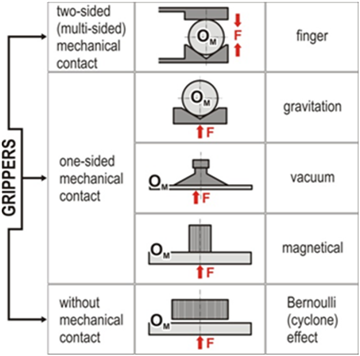• Figure 1. Types of the grippers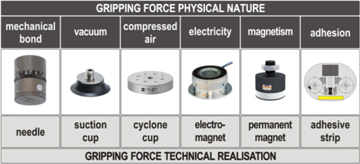• Figure 2. Unilateral (one-sided) gripping of the object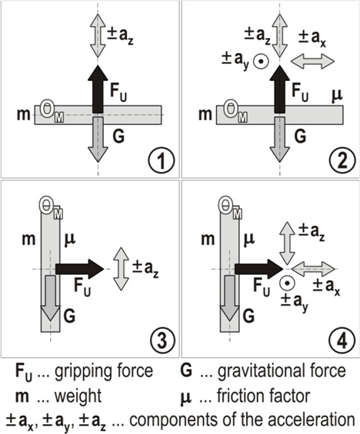• Figure 3. Possible manners of handling accelerations acting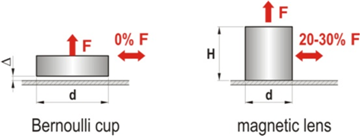• Figure 4. Conditions of grasping forces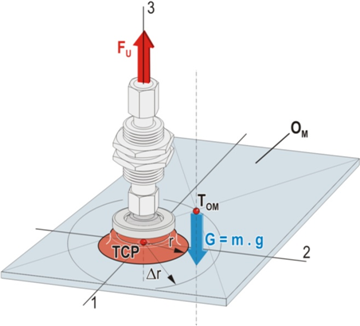• Figure 5. The ratios at the asymmetrical one-sided grasping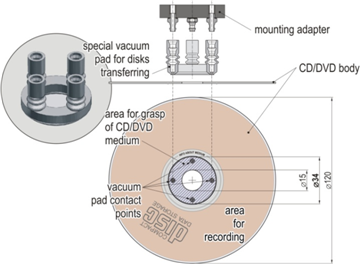• Figure 6. Example of area for grasping (manipulation with CD or DVD)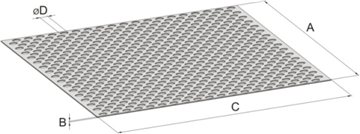• Figure 7. Perforated sheet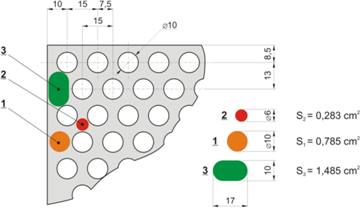• Figure 8. Conditions for vacuum suction cup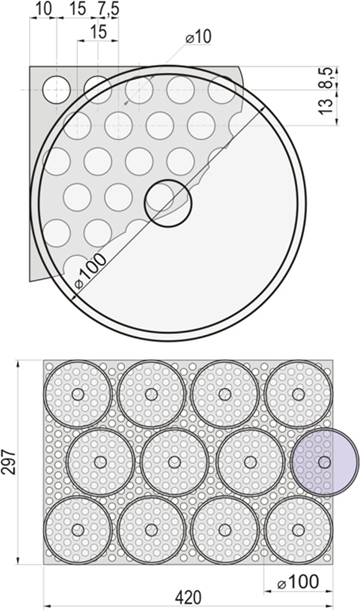• Figure 9. Conditions for Bernoulli suction cup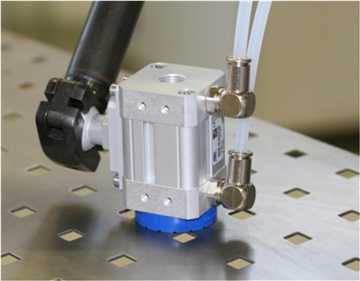• Figure 10. The gripping element having a permanent magnet (SCHMALZ)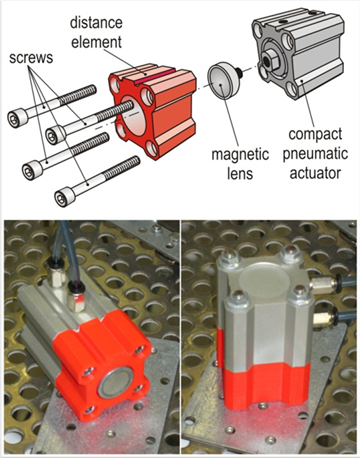• Figure 11. Gripping element on permanent magnet base KRME01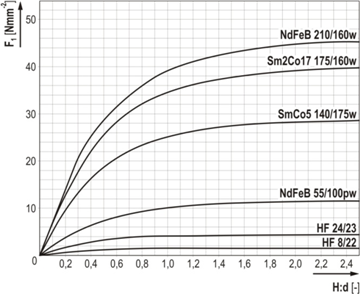• Figure 12. Gripping force of permanent magnets